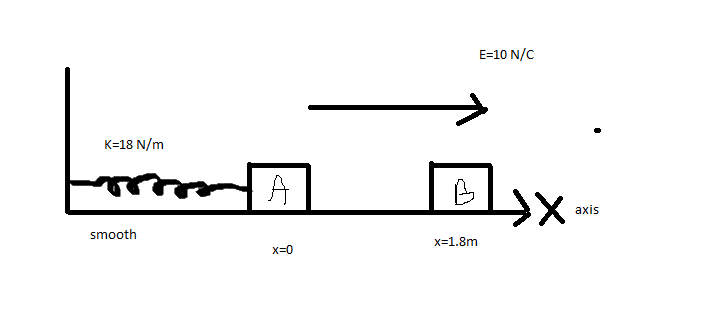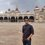# Amplitude of Oscillation!In the figure $m_A=m_B=1kg$. Block $A$ is neutral while $q_B=-1C$. sizes of $A$ and $B$ are negligible. $B$ is released from rest at a distance $1.8m$ from $A$. Initially springs is neither compressed nor elongated. The amplitude of oscillation of the combined mass will be?6 years, 3 months ago

This discussion board is a place to discuss our Daily Challenges and the math and science related to those challenges. Explanations are more than just a solution — they should explain the steps and thinking strategies that you used to obtain the solution. Comments should further the discussion of math and science.

When posting on Brilliant:

• Use the emojis to react to an explanation, whether you're congratulating a job well done , or just really confused .
• Ask specific questions about the challenge or the steps in somebody's explanation. Well-posed questions can add a lot to the discussion, but posting "I don't understand!" doesn't help anyone.
• Try to contribute something new to the discussion, whether it is an extension, generalization or other idea related to the challenge.

MarkdownAppears as
*italics* or _italics_ italics
**bold** or __bold__ bold
- bulleted- list
• bulleted
• list
1. numbered2. list
1. numbered
2. list
Note: you must add a full line of space before and after lists for them to show up correctly
paragraph 1paragraph 2

paragraph 1

paragraph 2

[example link](https://brilliant.org)example link
> This is a quote
This is a quote
    # I indented these lines
# 4 spaces, and now they show
# up as a code block.

print "hello world"
# I indented these lines
# 4 spaces, and now they show
# up as a code block.

print "hello world"
MathAppears as
Remember to wrap math in $$ ... $$ or $ ... $ to ensure proper formatting.
2 \times 3 $2 \times 3$
2^{34} $2^{34}$
a_{i-1} $a_{i-1}$
\frac{2}{3} $\frac{2}{3}$
\sqrt{2} $\sqrt{2}$
\sum_{i=1}^3 $\sum_{i=1}^3$
\sin \theta $\sin \theta$
\boxed{123} $\boxed{123}$

Sort by:

Block $B$ experiences a force of $F = q_B E = -10 \ \text{N}$ over a distance of $\Delta x = -1.8 \ \text{m}$.

So, it's velocity just before it hits Block $A$ is $v_B = -\sqrt{\dfrac{2 F \Delta x}{m_B}} = -6 \ \text{m/s}$.

Once Block $A$ and Block $B$ collide, their combined mass is $m_A+m_B$.

By conservation of momentum, the velocity of the combined mass just after collision is: $v_0 = \dfrac{m_B}{m_A+m_B}v_B = -3 \ \text{m/s}$.

The total force acting on Block $A$ and Block $B$ is $-kx+q_B E$ (spring force plus electric field force).

Using Newton's 2nd Law, the position of the combined mass as a function of time is given by the differential equation

$(m_A+m_B)x'' = -kx + q_B E$, i.e. $(2 \ \text{kg})x'' + (18 \ \text{N/m})x + 10 \ \text{N} = 0$,

with initial conditions $x(0) = 0 \ \text{m}$ and $x'(0) = -3 \ \text{m/s}$.

The solution is $x(t) = (0.556 \ \text{m})\cos\left((3 \ \text{rad/s})t\right) - \left(1 \ \text{m}\right)\sin\left((3 \ \text{rad/s})t\right) - (0.556 \ \text{m})$.

The amplitude of oscillation is simply $\sqrt{(0.556 \ \text{m})^2 + (-1 \ \text{m})^2} = 1.144 \ \text{m}$.

Let me know if there are any mistakes.

- 6 years, 3 months ago

thanks! I did it using W.E. Theorem.

- 6 years, 3 months ago

You have probably done no mistake. Here is an easier one: Equillibrium position is $x = \frac{-5}{9} , v_{x = 0} = 3m/s$ Applying conservation of energy from $x = 0 to x = \frac{-5}{9}$, $\frac{1}{2} (m_{A} + m_{B})((v_{max})^{2} - (v_{x=0})^{2}) +\frac{1}{2}kx^{2} = Ex$ Solving, we get $v_{max} = \frac{\sqrt{106}}{3}$ , Now, $A = \frac{v_{max}}{w} = \frac{\frac{\sqrt{106}}{3}}{3}$[as w = angular frequency =$3 \frac{rad}{sec}$] = $1.144 m$

- 6 years, 3 months ago

Can you please tell how to write in different paragraph, even the lines in different paras appear together.

- 6 years, 3 months ago

You have not mentioned anything about the nature of collision i.e. the value of coefficient of restitution for the collision to take place. Anyway, assuming it is a perfectly elastic collision, find the energy of the system just before collision and at either of compressed or elongated states. From there get the compression or elongation and your amplitude is double that value. ( The value of k is not visible enough, looks to be 16N/m , amplitude comes out to be 3m)

- 6 years, 3 months ago

The collision is perfectly inelastic, and $K=18 N/m$

- 6 years, 3 months ago

What is projectiles? A projectile is fired at an angle of 60 degre,to the horizontal and with the initial velocity of 80m/s.calculate A) time of flight B) the time taken to get to the maximum hight C) the rane D) the maximum range E) the velocity projection 2seconds after being fired(g=10m/s

- 6 years, 3 months ago

http://en.wikipedia.org/wiki/Projectile_motion

- 6 years, 3 months ago